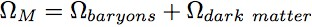Original Source

..

Dynamics of the Universe

Doctor James Schombert
Lecture #22, May 17, 2004
Physics Department
University of Oregon

..
Density of the Universe:

 the future of the Universe is determined if we are in an open or closed scenario the amount of mass is an important parameter to an open/closed Universe, but not the only parameter There are two possible futures for our Universe, continual expansion (open and flat), or turn-around and collapse (closed). Note that flat is the specific case of expansion to ever slowly speeds aproaching zero velocity. .. One of the key factors that determines which history is correct is the amount of mass/gravity for the Universe as a whole. If there is sufficient mass, then the expansion of the Universe will be slowed to the point of stopping, then retraction to collapse. If there is not a sufficient amount of mass, then the Universe will expand forever without stopping. The flat Universe is one where there is exactly the balance of mass to slow the expansion to zero, but not for collapse.

..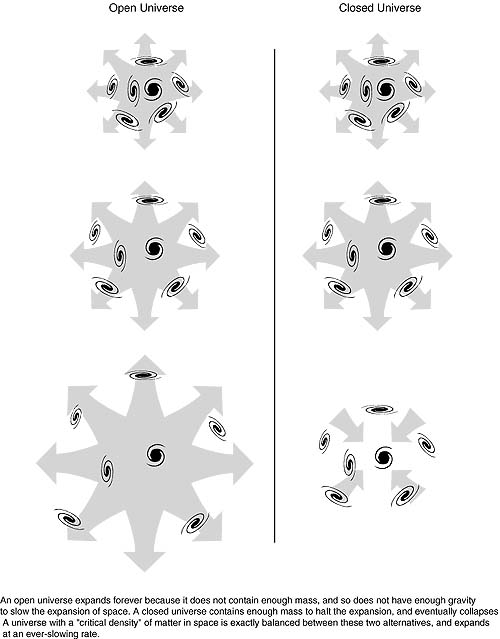.. the radius-time diagram displays, graphical fashion, the future of the Universe gravity, i.e. matter, determines the rate of expansion .. The future of the Universe is usually dipicted in a radius versus time diagram. Here the `radius', or scale factor, of the whole Universe is shown with respect to time. Regardless of the overall curvature of the Universe, we can charactersize its size with a scale factor in order to measure the change in spacetime as the Universe expands. A closed Universe will expand to some maximum size than contract back to zero. A flat or open Universe will increase in size forever. .. Our position in this diagram must be near the origin since it is difficult to directly determine which type of Universe we currently live in (at later times it becomes easier). Notice that since the Universe is currently 14 billion years, the time axis is measured in very long timescales. Hubble's constant measures the expanision rate of the Universe as it is today, thus is the slope of the radius-time diagram for time=today. If the Universe were empty, i.e. no matter, then the expansion rate would remain unchanged with time. The existence of matter, and therefore gravity, causes all of the possible Universes to slow down with time.

..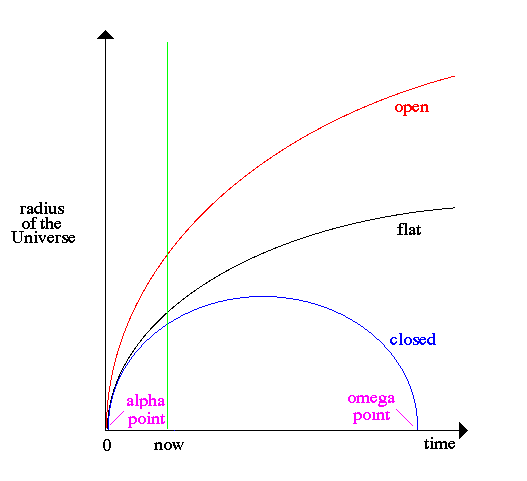.. modern cosmology is a search for two numbers, Ho and qo .. The parameter used to measure the rate of change in the expansion rate of the Universe is called qo, the deaccelration parameter (although, under the right conditions, qo can be positive and the Universe acceraltes). Knowledge of Ho and qo are the true goals of observational cosmology, for with both parameters it is possible to extract distances and ages of all things in the observable Universe.

..
Cosmological Models:

In modern cosmology, the different classes of Universes (open, flat or closed) are known as Friedmann universes and described by a simple equation:
..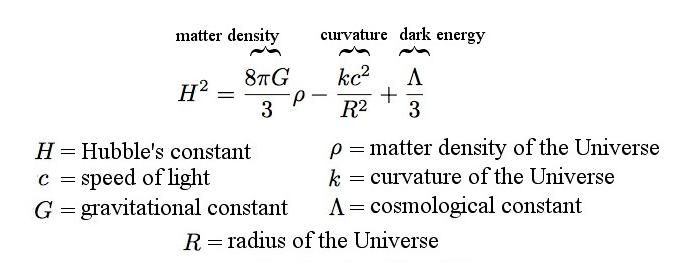..
In this equation, `R' represents the scale factor of the Universe (think of it as the radius of the Universe in 4D spacetime), and H is Hubble's constant, how fast the Universe is expanding. Everything in this equation is a constant, i.e. to be determined from observations. These observables can be broken down into three parts gravity (matter density), curvature and pressure or negative energy given by the cosmological constant.
..
Historically, we assumed that gravity was the only important force in the Universe, and that the cosmological constant was zero. Thus, if we measure the density of matter, then we could extract the curvature of the Universe (and its future history) as a solution to the equation. New data has indicated that a negative pressure, or dark energy, does exist and we no longer assume that the cosmological constant is zero.
..
Each of these parameters can close the Universe in terms of turn-around and collapse. Instead of thinking about the various constants in real numbers, we perfer to consider the ratio of the parameter to the value that matches the critical value between open and closed Universes. For example, the density of matter exceeds the critical value, the Universe is closed. We refer to these ratios as Omega (subscript M for matter, k for curvature, Lambda for the cosmological constant). For various reasons due to the physics of the Big Bang, the sum of the various Omega must equal one. And for reasons we will see in a later lecture, the curvature Omega is expected to be zero, allowing the rest to be shared between matter and the cosmological constant.
..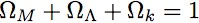..
The search for the value of matter density is a much more difficult undertaking. The luminous mass of the Universe is tied up in stars. Stars are what we see when we look at a galaxy and it fairly easy to estimate the amount of mass tied up in stars, gas, planets and assorted rocks. This is contains an estimate of what is called the baryonic mass of the Universe, i.e. all the stuff made of baryons = protons and neutrons. When these numbers are caluclated it is found that W for baryons is only 0.02, a very open Universe. However, when we examine motion of objects in the Universe, we quickly realize that most of the mass of the Universe is not seen, i.e. dark matter, which makes this estimate of W to be much too low. So we must account for this dark matter in our estimate.
..